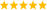# LabVIEW

cancel
Showing results for
Did you mean:

Highlighted

## Inflexion point and tangent

Hello,

This looks like a simple problem but nobody around found a way. I know this is possible to do because this is an exercice asked by a teacher who told me he succeded.

I have a number of plots in the graph that looks like this and I need to get the inflexion point and find the tangent of the inflexion point and then finds different elements on the graph.

how can labview handle these kind of things ? Thanks so much

Message 1 of 6
(158 Views)
Highlighted

## Re: Inflection point and tangent

"Inflection"

Think of the mathematical definitions of tangent and also inflection.

In your picture, you have a big black X and that says inflection point, but I really don't think that is the inflection pint.  I'm guessing you are looking for the point where it breaks from the flat line to the curve.

If you look at the "rate of change" of the Y value versus X, that will also give you the tangent.  It will also give you clues as to where the inflection point is.  (The rate of change of the rate of change will show a discontinuity.)

Message 2 of 6
(154 Views)
Highlighted

## Re: Inflexion point and tangent

Hi Oxbow,

@Oxbow8 wrote:

I have a number of plots in the graph that looks like this and I need to get the inflexion point and find the tangent of the inflexion point and then finds different elements on the graph.

how can labview handle these kind of things ?

LabVIEW can handle this for you: you need to apply the math on your data!

(Any other programming language requires the very same math…)

Best regards,
GerdWusing LV2011SP1 + LV2017 (+LV2019 sometimes) on Win10+cRIO
Message 3 of 6
(150 Views)
Highlighted

## Re: Inflexion point and tangent

I assume "inflection point". (inflexion point is apparently British spelling)

This is trivial given pure math but can be nearly impossible for discrete or noisy data unless you have a mathematical model for the curve. The inflection point could be anywhere in-between the discrete points and if there is noise you could have hundreds of inflection points. Right?

Where does the data come from? What's the mathematical formula for the curve? Looks like a damped response to a step function so maybe it's just a constant followed by an exponential.

@Oxbow8 wrote:

Hello,

This looks like a simple problem but nobody around found a way. I know this is possible to do because this is an exercice asked by a teacher who told me he succeded.

Your teacher is not nobody and I am sure he knows. 🙂

@Oxbow8 wrote:

... and then finds different elements on the graph.

All elements on the graph are "different", so you already found them.

@Oxbow8 wrote:

how can labview handle these kind of things ? Thanks so much

As has been said, this is not a LabVIEW problem, but a math problem. Translating it into any programming language, including LabVIEW, is trivial once you get the math right. What have you tried?LabVIEW Champion. It all comes together inGCentralWhat does "Engineering Redefined" mean??
Message 4 of 6
(93 Views)
Highlighted

## Re: Inflexion point and tangent

Hello all,

Yes sorry, inflexion = inflection

I created a .vi simulation file to try to solve this. Actually the inflection point is the beginning of the problems lol, I jointed a picture that explain everything I should get from a step response. I need the inflection point, the slope of the tangent at the inflection point, T1, T2, and Δy. This is more clear on the picture.

Ok... so maybe I should start doing a double derivative of the whole thing. I imagine by example a for loop that asks if the last x numbers are stables (=the difference is below a certain amount) of the derivative. the inflection point could be found when it's zero.

Message 5 of 6
(46 Views)
Highlighted

## Re: Inflexion point and tangent

@Oxbow8 wrote:

the inflection point is the beginning of the problems lol, I jointed a picture that explain everything I should get from a step response. I need the inflection point, the slope of the tangent at the inflection point, T1, T2, and Δy. This is more clear on the picture.

Ok... so maybe I should start doing a double derivative of the whole thing. I imagine by example a for loop that asks if the last x numbers are stables (=the difference is below a certain amount) of the derivative. the inflection point could be found when it's zero.

just do one (backward) derivative of the Y waveform, the point "I" should match with the maximum of the derived waveform

what does happen if you derive forward?

Message 6 of 6
(29 Views)